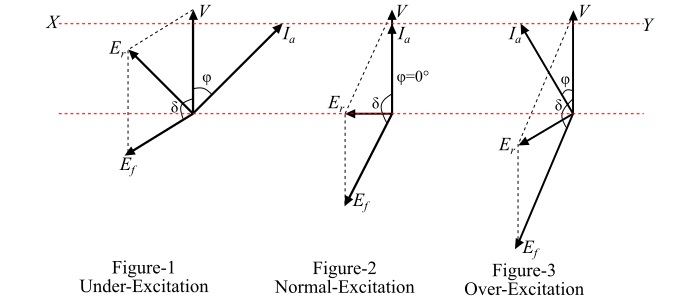# Effect of Changing Field Excitation on Synchronous Motor at Constant Load

One of the most important characteristics of a synchronous motor is that, by changing the field excitation of the motor, its power factor can be made both lagging and leading. The change in the power factor of the synchronous motor with the change in excitation can be explained with the help of its phasor diagram.

Consider a synchronous motor having a constant supply voltage and driving a constant mechanical load. The input power to the motor is given by,

$$\mathrm{P_{i}=\frac{VE_{f}}{X_{s}}Sinδ=3V\:I_{a}\:Cos\varphi}$$

Since V and XS are constant for a given synchronous motor, thus for constant power output,

$$\mathrm{E_{f}\:Sinδ=Constant}$$

$$\mathrm{I_{a}\:Cos\varphi=Constant}$$

When the field excitation (Ef) of the motor is changed, the armature current (Ia) changes. But the product $(I_{a}\:cos\varphi)$ must remain constant. For this to happen, the power factor angle $(\varphi)$ adjusts itself in such a way that product $(I_{a}\:cos\varphi)$ remains constant. That is, the power factor of the motor will change. For $(I_{a}\:Cos\varphi)$ to remain constant, the locus (represented by line XY) of tip of the phasor Ia must trace a straight line perpendicular to the phasor V (see the phasor diagrams shown in the figure).Form the figure, it is clear that as the value of excitation (Ef) increases, the magnitude of the armature current (Ia) first decreases and then increases again. The armature current is minimum at unity power factor and more at lagging or leading power factors.

## Case 1 − Under-Excitation of Synchronous Motor

The synchronous motor is said to be under-excited if the field excitation is such that (Ef<V). Under this condition, the armature current (Ia) lags behind the supply voltage (V) and consumes lagging reactive power (Q) i.e. the motor power factor is lagging as shown in Figure-1.

## Case 2 − Normal Excitation of Synchronous Motor

If (Ef=V), the synchronous motor is said to be normally excited. Under this condition, the reactive power of the motor is zero (i.e., Q = 0), that is the motor is neither absorbing nor delivering reactive power. Thus, the power factor of the motor is unity. For a given load, at unity power factor, the resultant voltage (Er) and hence, the armature current (Ia) are minimum as shown in Figure-2.

## Case 3 − Over-Excitation of Synchronous Motor

The synchronous motor is said to be over-excited if the field excitation is such that (Ef>V). Under such conditions, the armature current (Ia) leads the supply voltage (V) and the motor supplies lagging reactive power to the system. Hence, motor power factor is leading as shown in Figure-3.

## Conclusion

From the above discussion, it is concluded that,

• If the synchronous motor is under-excited, it has a lagging power factor.

• If the synchronous motor is normally-excited, it has unity power factor.

• If the synchronous motor is over-excited, it has a leading power factor.